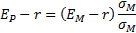/

### Enterprise Risk Management Formula Book 10. Portfolio optimisation

If there areasset categories then a (one period) mean-variance efficient portfolio,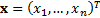where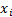is the amount (or weight) invested in the’th asset, given a benchmark,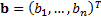, assumed future (one period) mean returns  on each asset,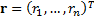, and an assumed covariance matrix between the (one period) returns on different assets,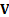, is a portfolio that maximises the utility function,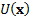for some risk aversion parameter,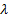, subject to relevant constraints on the, where: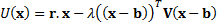The constraints that are applied are usually linear, i.e. of the form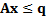which if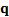is an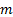dimensional vector is understood as meaning that there areconstraints each of the form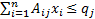. In such a formulation, equality constraints, including that the amounts invested add up to the total portfolio value (or the weights add to unity), can be written as two inequality constraints, e.g.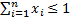and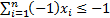combined.

The implied alphas with a mean-variance risk-return model given a portfolio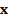and benchmark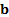are the mean returns that need to be assumed for the different asset categories forto be mean-variance optimal for some value of. They can only meaningfully be determined for assets whose weights in the portfolio are not constrained (other than by the constraint that weights add to unity). They are then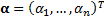where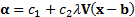where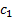and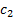are arbitrary scalar constants.

10.2        Capital Asset Pricing Model (CAPM)

The security market line is: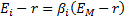where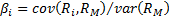The capital market line (for efficient portfolios) is: